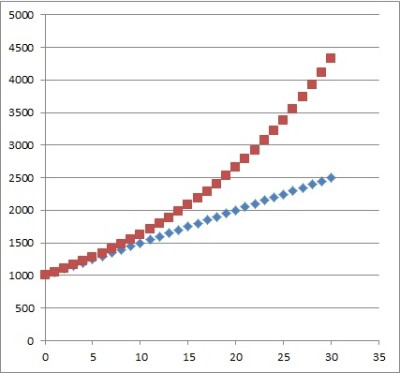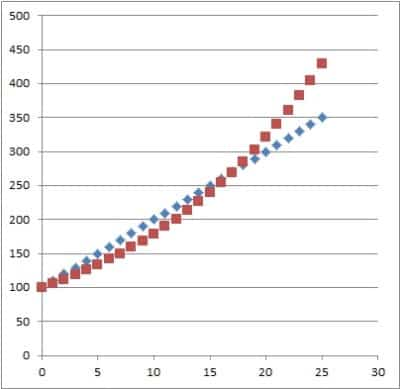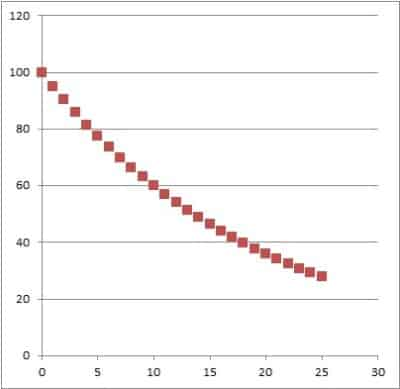# Simple Interest Vs Compound Interest (3 Key Concepts)

Interest rates are an important topic when dealing with anything related to finances, including loans and investments.  However, we still need to make the distinction between simple and compound interest.

So, what is the difference between simple interest and compound interest?  An account paying simple interest increases by a fixed amount in each time period (linear growth). An account paying compound interest increases by a fixed percentage in each time period (exponential growth). Compound interest always outgrows simple interest eventually (assuming positive rates).

Of course, when negative interest rates are negative, an account with compound interest will decrease faster than an account with simple interest.

In this article, we’ll take a look at the key difference between simple interest and compound interest.  We’ll also look at some examples and answer some common questions about these topics.

Let’s get started.

## Simple Interest Vs Compound Interest

Simple interest and compound interest tell us how the value of an account grows over time.

An account paying simple interest grows by a fixed amount in each time period (usually a day, week, month, or year).  If we graph the balance of an account paying simple interest, we will see a linear function (more on this below).

An account paying compound interest grows by a fixed percentage of the previous value (and thus, an increasing amount) in each time period.  If we graph the balance of an account paying compound interest, we will see an exponential growth function (more on this below).

*Note: for negative interest rates, the graph would be an exponential decay function.

### Formulas For Simple Interest & Compound Interest

For an account paying simple interest, the formula is given by:

• A(t) = A0(1 + rt)

or

• A(t) = A0 + A0rt [A0 is the starting account balance, and A0rt is the accumulated interest]

where:

• A(t) is the account balance at time t
• A0 is the starting account balance
• r is the interest rate on the account (paid per time period t)
• t is the number of time periods that have passed

For an account paying compound interest, the formula is given by:

• A(t) = A0(1 + r)t

where:

• A(t) is the account balance at time t
• A0 is the starting account balance
• r is the interest rate on the account (paid per time period t)
• t is the number of time periods that have passed

### Differences Between Simple Interest & Compound Interest

You can see the graphs of a simple interest account (blue) and a compound interest account (red) in the picture below.  The relevant values are:

• A0 = \$1,000 (the starting account balance)
• r = 5%, or 0.05 (the annual interest rate on the account)
• t is counted in yearsThis graph shows simple interest (blue dots) and compound interest (red dots) over 30 years with a starting balance of \$1,000 and an annual interest rate of 5%.

The table below summarizes the differences between simple interest and compound interest side-by-side, so you can compare them at a glance.

Now let’s look at how to calculate simple interest.

### How To Calculate Simple Interest

To calculate simple interest, we need to use one of the formulas for simple interest, use the known values as inputs, and solve for the unknown value.

Let’s take a look at some examples now.

#### Example 1: How To Calculate Simple Interest (Finding The Annual Interest)

Let’s say that you have an account that pays simple interest with a starting balance of \$100 and an annual interest rate of 2%.  To find the annual interest (how much the account grows by per year), we use the formula:

• (Annual Simple Interest) = A0rt
• (Annual Simple Interest) = \$100(0.02)(1)  [t = 1 since the time period is 1 year for an annual rate]
• (Annual Simple Interest) = \$2

If the account were paying 2% per month instead of 2% per year, then there would be t = 12 periods in one year, and the calculation would change as follows:

• (Annual Simple Interest) = A0rt
• (Annual Simple Interest) = \$100(0.02)(12)  [t = 11 since there are 12 months in a year]
• (Annual Simple Interest) = \$24

This is equivalent to an annual simple interest rate of \$24/\$100 = 0.24 or 24%.

#### Example 2: How To Calculate Simple Interest (Finding The Interest Rate)

Let’s say that you have an account that pays simple interest of \$48 per year with a starting balance of \$200.  To find the annual interest rate we use the same formula as above:

• (Annual Simple Interest) = A0rt
• \$48 = \$200r(1)
• 0.24 = r

So the simple interest rate is 24% per year.

If the account paid out interest every month instead of every year, we would use t = 12 (months in a year) and the calculation would change as follows:

• (Annual Simple Interest) = A0rt
• \$48 = \$200r(12)
• 0.02 = r

So the simple interest rate is 2% per month.

#### Example 3: How To Calculate Simple Interest (Finding The Principal Amount)

Let’s say that you have an account that pays simple interest of \$36 per year with an interest rate of 12%.  To find the starting balance, we use the same formula as above:

• (Annual Simple Interest) = A0rt
• \$36 = A0(0.12)(1)
• \$36/0.12 = A0
• \$300 = A0

So, the starting account balance is \$300.

#### Example 4: How To Calculate Simple Interest (Finding The Final Balance)

Let’s say that you have an account that pays simple interest with a starting balance of \$500 and an annual interest rate of 6%.  To find the final balance after 10 years, we use the simple interest formula we gave earlier:

• A(t) = A0(1 + rt)
• A(10) = \$500(1 + (0.06)(10))
• A(10) = \$500(1 + (0.6))
• A(10) = \$500(1.6)
• A(10) = \$800

So, this account with simple interest would have \$800 after 10 years.

### How To Calculate Compound Interest

To calculate compound interest, we need to use the formula for compound interest, use the known values as inputs, and solve for the unknown value.

Let’s take a look at some examples now.

#### Example 1: How To Calculate Compound Interest (Finding The Annual Interest)

Let’s say that you have an account that pays compound interest with a starting balance of \$100 and an annual interest rate of 3%.  To find the annual interest (how much the account grows by per year), we will set up a table and use the formula for compound interest to fill it in.

Filling in the starting balance A0 = \$100 and the interest rate r = 0.03 (3%), we get:

• A(t) = A0(1 + r)t
• A(t) = \$100(1 + 0.03)t
• A(t) = \$100(1.03)t

After t = 1 year:

• A(t) = \$100(1.03)1
• A(t) = \$103

After t = 2 years:

• A(t) = \$100(1.03)2
• A(t) = \$100(1.0609)
• A(t) = \$106.09

After t = 3 years:

• A(t) = \$100(1.03)3
• A(t) = \$100(1.092727)
• A(t) = \$109.27

and so forth for t = 4, 5, 6, …

Setting up our table, we get:

[table with year, amount, difference = annual interest]

#### Example 2: How To Calculate Compound Interest (Finding The Annual Interest Rate)

Let’s say that you have an account that pays compound interest with a starting balance of \$100 and an ending balance of \$146.41 after 4 years.  To find the annual interest rate, we will use the formula for compound interest:

• A(t) = A0(1 + r)t
• A(4) = \$100(1 + r)4  [starting balance of A0 = \$100, t = 4 years, r is unknown]
• \$146.41 = \$100(1 + r)4  [ending balance of A(4) = \$146.41]
• \$146.41/\$100.00 = (1 + r)4
• 1.4641 = (1 + r)4
• (1.4641)1/4 = 1 + r
• 1.1 = 1 + r
• 0.1 = r

So r = 0.1, meaning that the account pays a 10% annual compound interest rate.

#### Example 3: How To Calculate Compound Interest (Finding The Principal Amount)

Let’s say that you have an account that pays compound interest of 20% per year with an ending balance of \$4,976.64 after 5 years.  To find the principal amount (starting balance), we will use the formula for compound interest:

• A(t) = A0(1 + r)t
• A(5) = A0(1 + 0.2)5  [starting balance unknown, t = 5 years, r = 0.2 (20%)]
• \$4,976.64 = A0(1.2)5  [ending balance of A(5) = \$2.488.32]
• \$4,976.64 = A0(2.48832)
• \$4,976.64/2.48832 = A0
• \$2,000 = A0

So A0 = \$2,000 is the starting balance for this account that bears compound interest.

#### Example 4: How To Calculate Compound Interest (Finding The Final Balance)

Let’s say that you have an account that pays compound interest of 5% per year with a starting balance of \$3,000.  To find the final balance after 3 years we will use the formula for compound interest:

• A(t) = A0(1 + r)t
• A(5) = \$3,000(1 + 0.05)3  [starting balance of A0 = \$3,000, t = 3 years, r = 0.05 (5%)]
• A(5) = \$3,000(1.05)3
• A(5) = \$3,000(1.157625)
• A(5) = \$3,472.88

So A(5) = \$3,472.88is the final balance for this account that bears compound interest.

### Does Simple Interest Compound?

Simple interest does not compound.  Any interest that is paid is added to the account, but it is not added to the principal.

In other words, to calculate the amount of interest paid each time period, we take a fixed percentage (the interest rate) of a fixed value (the starting balance).

### Does Simple Interest Grow Exponentially?

Simple interest does not grow exponentially.  Instead, simple interest grows in a linear fashion.

This is because the amount of interest earned is the same in each time period.  The amount of interest earned is the “slope” of the line.

Remember the 2nd simple interest formula from earlier:

• A(t) = A0 + A0rt

If we treat t as the “x” variable and A(t) as the “y” variable, then the slope is m = A0r and the y-intercept is b = A0:

• y = mx + b

### Can Compound Interest Be Equal To Simple Interest?

Compound interest can be equal to simple interest at a certain point in time.  To figure out when this occurs, we would need to solve the equation that sets them equal:

• (Simple Interest Account Value) = (Compound Interest Account Value)
• A0(1 + rt) = A0(1 + r)t
• (1 + rt) = (1 + r)t

We can see that the two sides of this equation will be equal when t = 0 and t = 1. There are no other solutions.

To see this, consider the other possible values of t.

For an integer t greater than 1, the right side will have the first two terms 1 + rt. After subtracting 1 + rt from both sides, the left side of the equation would be zero, and the right side would be positive (since r is positive). So, there are no other solutions to the equation.

#### Can Simple Interest Exceed Compound Interest?

Technically, the graph of simple interest is “above” the graph of compound interest between t = 0 and t = 1 (when both have the same interest rate r, the same starting balance A0, and the same compounding period).

However, since we are dealing with discrete time periods, this will never actually happen.

For t > 1, compound interest will exceed simple interest.

Of course, if the simple interest rate is higher than the compound interest rate, then simple interest can exceed compound interest for many time periods (but compound interest will always catch up eventually).

For example, with a starting balance of \$100, a simple interest account paying 10% starts off ahead of a compound interest account paying 6%. However, the compound interest account catches up just after the 17th year and surpasses the simple interest account.The simple interest account paying 10% (blue) starts out ahead of the compound interest account paying 6% (red), but after year 17, compound interest catches up.

### Does Compound Interest Grow Exponentially?

Compound interest grows exponentially, meaning that it increases by percentage of the previous value (instead of growing by a fixed amount) in each time period.  We can see this from the equation for compound interest:

• A0(1 + r)t
• A0etlog(1+r)

In other words, a compound interest function has the form

• f(t) = aebt

where a = A0 (the starting balance) and b = log(1 + r).

### Can Compound Interest Be Negative?

Compound interest can be negative in some cases.  It sound strange, but it is true!

For example, some countries have (or had) negative interest rates (as set by their central banks), including:

• Switzerland
• Denmark
• Japan
• Sweden

Real interest rates can also be negative due to the effect of inflation, even if nominal interest rates are positive.

For example, if your stock portfolio returns 4% in a given year, but inflation is 9.2%, then your portfolio lost 5% of its value in real terms (after adjusting for inflation):

• 1.04/1.092 = 1/1.05 (5% inflation).

With a negative real interest rate, the graph of an account bearing compound interest would show exponential decay, as pictured below.This compound interest account pays a negative interest rate (-5%), so its graph displays exponential decay.

## Conclusion

Now you know about the differences between simple interest and compound interest.  You also know how to do some of the calculations to solve interest rate problems.